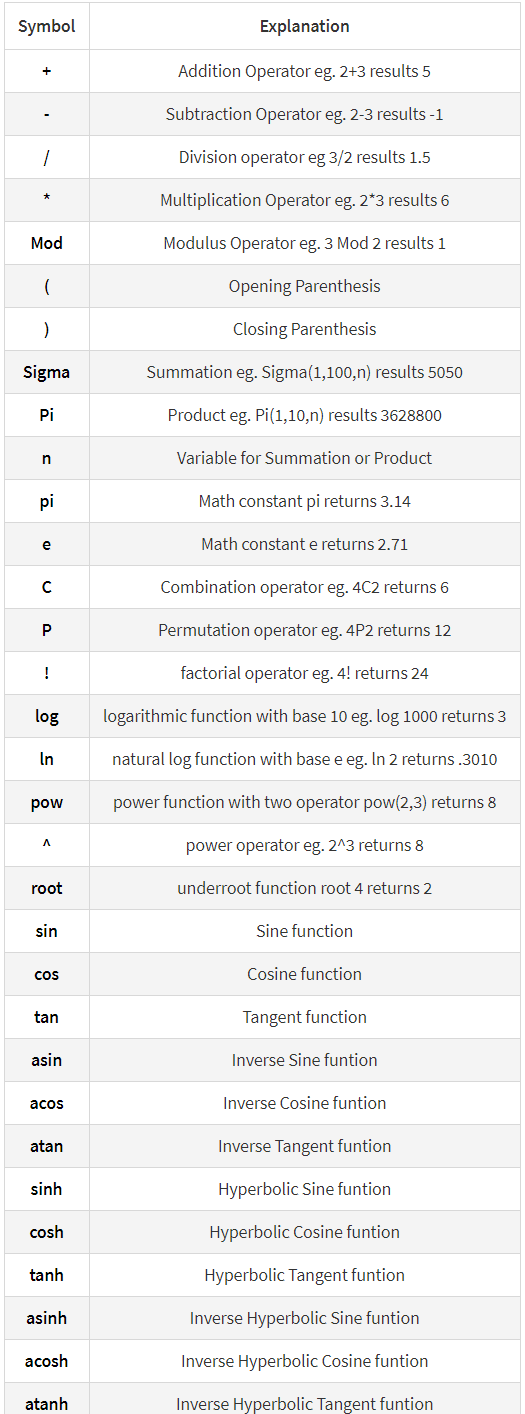# Download Elementor Form Cost Calculator on Codecanyon

\$25.00

## Description Elementor Form Cost Calculator :

Download Elementor Form Cost Calculator. This App releases on Tuesday 26th April 2022 By The author rednumber on Codecanyon. It’s uses with calculations,contact form,Cost Calculator,elementor,elementor Cost Calculator,elementor form,elementor number,elementor number format,elementor pro,wp forms.

Item Title: Elementor Form Cost Calculator
Category: wordpress/forms
Price: \$25
Author: rednumber
Published Date: Tuesday 26th April 2022 04:44:02 AM

## Elementor Form – Calculations

Create form calculators, advanced cost estimators extensive for Elementor Form

With this Add-on for Elementor form you can create advanced calculation forms based on the user input. Make awesome booking and order forms or create complex estimation forms. It is as easy as doing normal mathematics.

#### Conditional calculations

if(A < B,A + 1, A +3 ) + 5 + if(number1 < number2, number-600 + 15, 1) +7

#### Dates calculations

days( date2, date1 )
months( date2, date1 )
year( date2, date1 )

### EQUATIONS / FORMULAS FORMAT FOR CALCULATED FIELDS

With simple mathematical operations:

```sin(cos(tan(90))) * Sigma(1,15,n) + 2^3

fieldname1 / fieldname2

(fieldname1 * ( fieldname2 + fieldname3 ) ) / 5

fieldname1 * ( fieldname2 + fieldname3 )
```

## MAIN FEATURES

• Easy use
• Number and Total formats
• Supports conditional calculations
• Support all mathematical formulas
• Creating forms with automatically calculated fields
• Multi Total field
• Cross browser’s compatible
• Custom format total field
• Finance calculators
• Quote calculators
• Booking cost calculators
• And more…

### Supported symbols### CHANGELOG

```

13/Apr/2022: (version 0.1)
- Version 1.0 Initial Release
```

#Elementor #Form #Cost #Calculator

## User Reviews

0.0 out of 5
0
0
0
0
0

There are no reviews yet.

Download Elementor Form Cost Calculator on Codecanyon

\$25.00